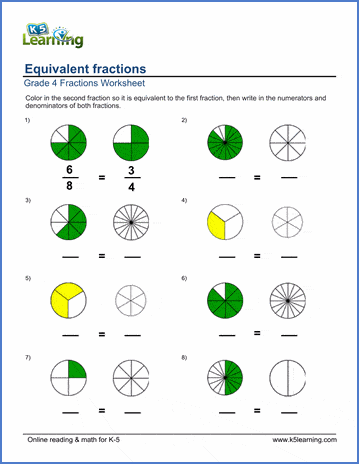# Coloring in equivalent fractions

## Equivalent fraction worksheets: coloring and naming equivalent fractions

Below are six versions of our grade 4 equivalent fractions worksheet; students are given a pie chart representing a fraction and must color in a 2nd pie chart (with a different number of slices) to create an equivalent fraction. Students are then asked to write the numerators and denominators of each fraction. These worksheets are pdf files.## More fractions worksheets

Explore all of our fractions worksheets, from dividing shapes into "equal parts" to multiplying and dividing improper fractions and mixed numbers.

## What is K5?

K5 Learning offers reading and math worksheets, workbooks and an online reading and math program for kids in kindergarten to grade 5.  We help your children build good study habits and excel in school.